# 1. 项目介绍

• Your first goal is to write code including a series of steps (pipeline) that identify and draw the lane lines on a few test images.
• Once you can successfully identify the lines in an image, you can cut and paste your code into the block provided to run on a video stream.
1. 处理一系列单独的图片-识别出车线并叠加红色线条，develop your pipeline on a series of individual images
2. 将上述的方法应用到video中，apply the result to a video stream (really just a series of images) - 输出结果类似raw-lines-example.mp4
3. 用插值的方式，将识别出来的车道线连接起来，get creative and try to average and/or extrapolate the line segments you’ve detected to map out the full extent of the lane lines - 输出结果类似P1_example.mp4
4. 写一个总结文档，there is a brief writeup to complete

1. writeup_template.md, 总结文档
2. P1.ipynb, notebook文件
3. examples文件夹，包含原始图片及处理后的图片，原始video和处理后的video
4. test_images文件夹，左行右行等各种原始图片
5. test_videos文件夹，三个原始的行驶video
6. test_videos_output文件夹，结果输出用的文件夹
• To find the lanes I used below steps as my pipeline:
• Converting image to grayscale
• Blurring an image by a Gaussian function
• Using CannyEdge to find the edges
• Applying the region of interest and keep only the edges which are present in Region of Interest
• Using hough algorithm to detect the lines from the image
• Adding the lane lines to the original image
• Creating two groups of lines based on the slope and also exclude lines that are not in the specific threshold.
• Using the points of left and right line get the slope and b of y = slope*x + b
• Calculating start and ends points to make lines with a straight line
• Calculating the means for creating the left lane and right lane

# 2. 识别车线

The tools you have are:

• color selection
• region of interest selection
• grayscaling
• Gaussian smoothing
• Canny Edge Detection
• Hough Tranform line detection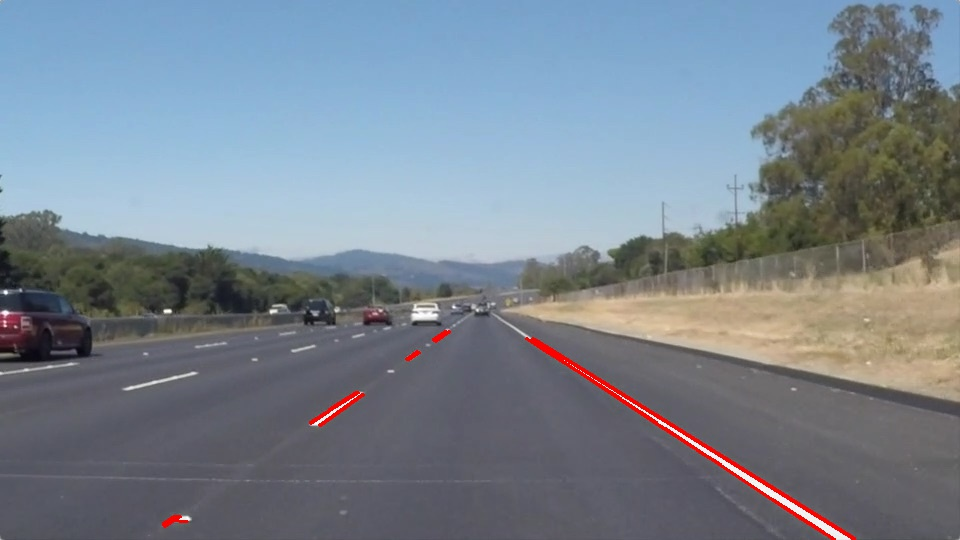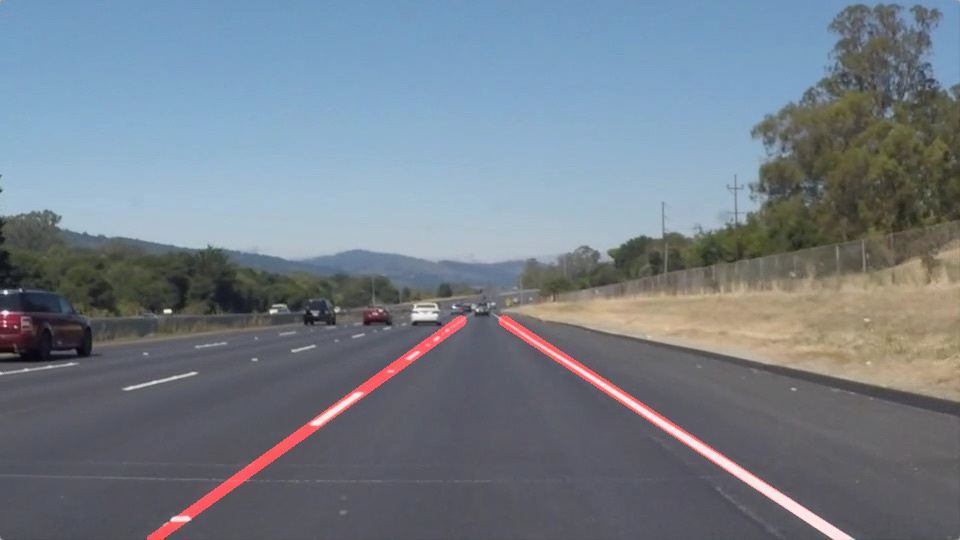# 3. helper functions介绍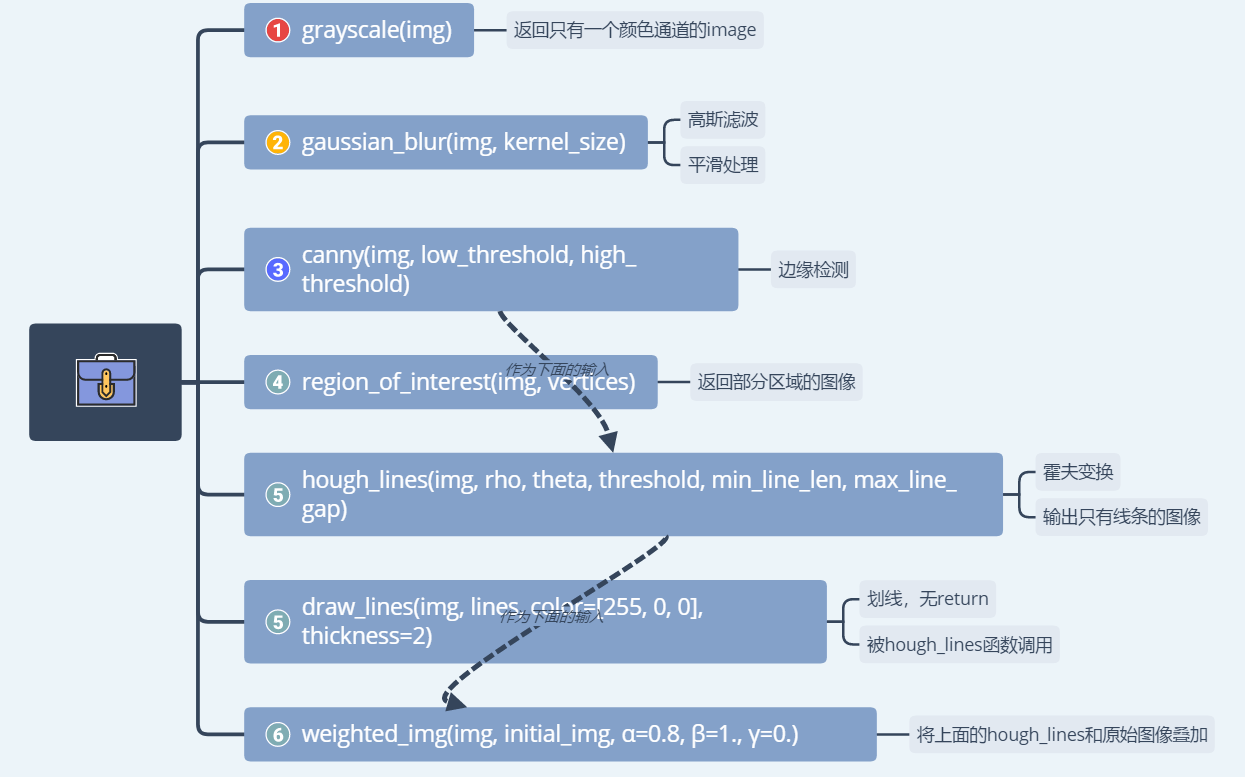# 4. 代码详细

## 4.1 Import Packages

``````#importing some useful packages
import matplotlib.pyplot as plt
import matplotlib.image as mpimg
import numpy as np
import cv2
%matplotlib inline
``````

## 4.2 Read in an Image

``````#reading in an image

#printing out some stats and plotting
print('This image is:', type(image), 'with dimensions:', image.shape)
plt.imshow(image)  # if you wanted to show a single color channel image called 'gray', for example, call as plt.imshow(gray, cmap='gray')
``````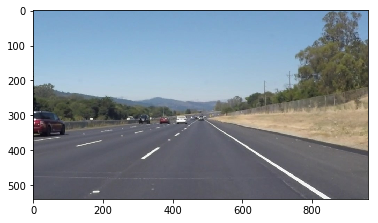## 4.3 Ideas for Lane Detection Pipeline

``````**Some OpenCV functions (beyond those introduced in the lesson) that might be useful for this project are:**

`cv2.inRange()` for color selection
`cv2.fillPoly()` for regions selection
`cv2.line()` to draw lines on an image given endpoints
`cv2.cvtColor()` to grayscale or change color
`cv2.imwrite()` to output images to file
`cv2.bitwise_and()` to apply a mask to an image

**Check out the OpenCV documentation to learn about these and discover even more awesome functionality!**
``````

## 4.4 Helper Functions

``````import math

def grayscale(img):
"""Applies the Grayscale transform
This will return an image with only one color channel
but NOTE: to see the returned image as grayscale
(assuming your grayscaled image is called 'gray')
you should call plt.imshow(gray, cmap='gray')"""
#plt.imshow(gray, cmap='gray')
return cv2.cvtColor(img, cv2.COLOR_RGB2GRAY)
# return cv2.cvtColor(img, cv2.COLOR_BGR2GRAY)

def canny(img, low_threshold, high_threshold):
"""Applies the Canny transform"""
return cv2.Canny(img, low_threshold, high_threshold)

def gaussian_blur(img, kernel_size):
"""Applies a Gaussian Noise kernel"""
return cv2.GaussianBlur(img, (kernel_size, kernel_size), 0)

def region_of_interest(img, vertices):
"""

Only keeps the region of the image defined by the polygon
formed from `vertices`. The rest of the image is set to black.
`vertices` should be a numpy array of integer points.
"""

#defining a 3 channel or 1 channel color to fill the mask with depending on the input image
if len(img.shape) > 2:
channel_count = img.shape  # i.e. 3 or 4 depending on your image
else:

#filling pixels inside the polygon defined by "vertices" with the fill color

#returning the image only where mask pixels are nonzero

def draw_lines_ori(img, lines, color=[255, 0, 0], thickness=7):
"""
NOTE: this is the function you might want to use as a starting point once you want to
average/extrapolate the line segments you detect to map out the full
extent of the lane (going from the result shown in raw-lines-example.mp4
to that shown in P1_example.mp4).

Think about things like separating line segments by their
slope ((y2-y1)/(x2-x1)) to decide which segments are part of the left
line vs. the right line.  Then, you can average the position of each of
the lines and extrapolate to the top and bottom of the lane.

This function draws `lines` with `color` and `thickness`.
Lines are drawn on the image inplace (mutates the image).
If you want to make the lines semi-transparent, think about combining
this function with the weighted_img() function below
"""

for line in lines:
for x1,y1,x2,y2 in line:
cv2.line(img, (x1, y1), (x2, y2), color, thickness)

def draw_lines(img, lines, color=[255, 0, 0], thickness=7):

#list to get positives and negatives values

x_bottom_pos = []
x_upperr_pos = []
x_bottom_neg = []
x_upperr_neg = []

y_bottom = 540
y_upperr = 315

slope = 0
b = 0

#get x upper and bottom to lines with slope positive and negative
for line in lines:
for x1,y1,x2,y2 in line:
#test and filter values to slope
if ((y2-y1)/(x2-x1)) > 0.5 and ((y2-y1)/(x2-x1)) < 0.8 :

slope = ((y2-y1)/(x2-x1))
b = y1 - slope*x1

x_bottom_pos.append((y_bottom - b)/slope)
x_upperr_pos.append((y_upperr - b)/slope)

elif ((y2-y1)/(x2-x1)) < -0.5 and ((y2-y1)/(x2-x1)) > -0.8:

slope = ((y2-y1)/(x2-x1))
b = y1 - slope*x1

x_bottom_neg.append((y_bottom - b)/slope)
x_upperr_neg.append((y_upperr - b)/slope)

#creating a new 2d array with means
lines_mean = np.array([[int(np.mean(x_bottom_pos)), int(np.mean(y_bottom)), int(np.mean(x_upperr_pos)), int(np.mean(y_upperr))],
[int(np.mean(x_bottom_neg)), int(np.mean(y_bottom)), int(np.mean(x_upperr_neg)), int(np.mean(y_upperr))]])

#Drawing the lines
for i in range(len(lines_mean)):
cv2.line(img, (lines_mean[i,0], lines_mean[i,1]), (lines_mean[i,2], lines_mean[i,3]), color, thickness)

def hough_lines(img, rho, theta, threshold, min_line_len, max_line_gap):
"""
`img` should be the output of a Canny transform.

Returns an image with hough lines drawn.
"""
lines = cv2.HoughLinesP(img, rho, theta, threshold, np.array([]), minLineLength=min_line_len, maxLineGap=max_line_gap)
line_img = np.zeros((img.shape, img.shape, 3), dtype=np.uint8)
draw_lines(line_img, lines)
return line_img

# Python 3 has support for cool math symbols.

def weighted_img(img, initial_img, α=0.8, β=1., γ=0.):
"""
`img` is the output of the hough_lines(), An image with lines drawn on it.
Should be a blank image (all black) with lines drawn on it.

`initial_img` should be the image before any processing.

The result image is computed as follows:

initial_img * α + img * β + γ
NOTE: initial_img and img must be the same shape!
"""
return cv2.addWeighted(initial_img, α, img, β, γ)
``````

## 4.5 Test Images

Build your pipeline to work on the images in the directory “test_images”
You should make sure your pipeline works well on these images before you try the videos.

``````import os
imgpath = os.listdir("test_images/")
print(imgpath)
``````

## 4.6 Applies the Grayscale transform/gaussian_blur/Canny transform

``````image = mpimg.imread('test_images/solidWhiteCurve.jpg')
color_select = np.copy(image)

# gray transform
gray = grayscale(color_select)

# Define a kernel size for Gaussian smoothing / blurring
kernel_size = 5
blur_gray = gaussian_blur(gray,kernel_size)

# Define parameters for Canny and run it
low_threshold = 100
high_threshold = 250
edges = canny(blur_gray, low_threshold, high_threshold)

# Display the image
plt.figure(figsize=(8,6))
plt.imshow(edges, cmap='Greys_r')
``````
``````image = mpimg.imread('test_images/solidWhiteCurve.jpg')
color_select = np.copy(image)

# gray transform
gray = grayscale(color_select)

# Define a kernel size for Gaussian smoothing / blurring
kernel_size = 5
blur_gray = gaussian_blur(gray,kernel_size)

# Define parameters for Canny and run it
low_threshold = 100
high_threshold = 250
edges = canny(blur_gray, low_threshold, high_threshold)

# Display the image
plt.figure(figsize=(8,6))
plt.imshow(edges, cmap='Greys_r')
``````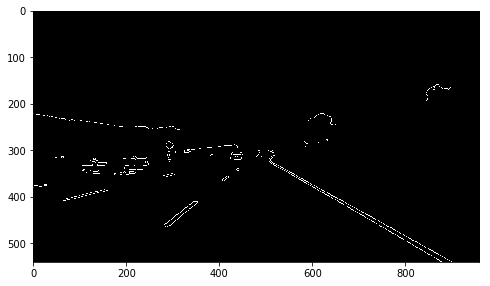## 4.7 Set a region

``````# This time we are defining a four sided polygon to mask
imshape = image.shape
left_bottom = (0,imshape) # 1
right_bottom = (imshape,imshape) # 4
right_top =  ((imshape/2)+50, (imshape/2 + 60)) # 3
left_top = ((imshape/2 )-50, (imshape/2 + 60)) # 2

vertices = np.array([[left_bottom,left_top,right_top, right_bottom]], dtype=np.int32)
``````
``````selectimg = region_of_interest(color_select,vertices)

plt.figure(figsize=(8,6))
plt.imshow(selectimg)
``````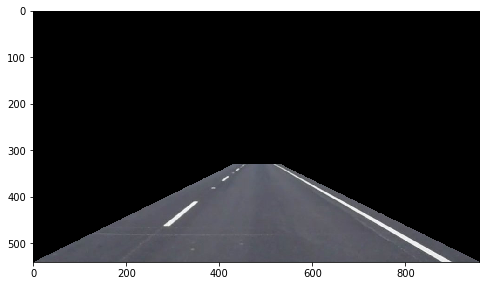``````selectedges = region_of_interest(edges,vertices)

plt.figure(figsize=(8,6))
plt.imshow(selectedges, cmap='Greys_r')
``````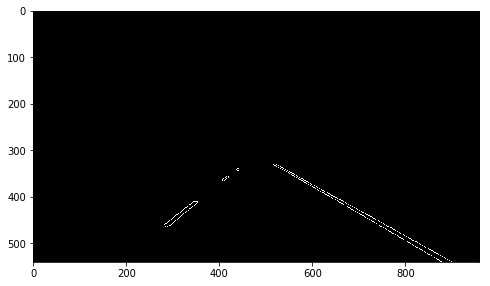## 4.8 Run Hough on edge detected image

``````rho = 1
theta = np.pi/90
threshold = 30
min_line_length = 40
max_line_gap = 400

lines = hough_lines(selectedges, rho, theta, threshold,min_line_length, max_line_gap)

plt.figure(figsize=(8,6))
plt.imshow(lines)
``````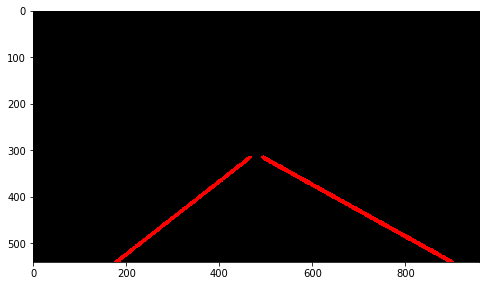## 4.9 Merge Lines with the original image

``````lines_edges = weighted_img(lines, image, α=0.8, β=1., γ=0.)

plt.figure(figsize=(8,6))
plt.imshow(lines_edges)
``````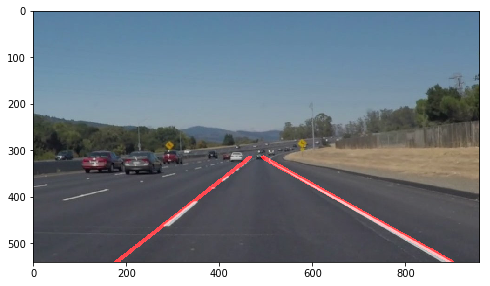## 4.10 Build a Lane Finding Pipeline

Build the pipeline

``````def pipeline_img(image,
kernel_size = 5,
low_threshold = 100, high_threshold=250,
rho=1, theta=np.pi/90, threshold=30,
min_line_len=40, max_line_gap=400):

color_select = np.copy(image)

# gray transform
gray = grayscale(color_select)

# Gaussian smoothing / blurring
blur_gray = gaussian_blur(gray, kernel_size)

#finding edges
edges = canny(blur_gray, low_threshold, high_threshold)

#setting vertices
imshape = image.shape
left_bottom = (0,imshape) # 1
right_bottom = (imshape,imshape) # 4
right_top =  ((imshape/2)+50, (imshape/2 + 60)) # 3
left_top = ((imshape/2 )-50, (imshape/2 + 60)) # 2

vertices = np.array([[left_bottom,left_top,right_top, right_bottom]], dtype=np.int32)

#creating a region of interest

#finding lines
lines = hough_lines(masked_edges, rho, theta, threshold, min_line_len, max_line_gap)

#merge with original image
lines_edges = weighted_img(lines, image, α=0.8, β=1., γ=0.)

return lines_edges
``````

** Processing the images and saving them to the ouput**

``````import os
imgname = os.listdir("test_images/")

for img in imgname:
imgpath = "test_images/" + img

image_processed = pipeline_img(image,
kernel_size = 5,
low_threshold = 100, high_threshold=250,
rho=1, theta=np.pi/90, threshold=30,
min_line_len=40, max_line_gap=400)
mpimg.imsave("test_images_output/" + img, image_processed)
``````

## 4.11 Test on Videos

``````# Import everything needed to edit/save/watch video clips

from moviepy.editor import VideoFileClip
from IPython.display import HTML

def process_image(image):
# NOTE: The output you return should be a color image (3 channel) for processing video below
# TODO: put your pipeline here,
# you should return the final output (image where lines are drawn on lanes)

result = pipeline_img(image,
kernel_size = 5,
low_threshold = 100, high_threshold=250,
rho=1, theta=np.pi/90, threshold=30,
min_line_len=40, max_line_gap=400)

return result

white_output = 'test_videos_output/solidWhiteRight.mp4'
## To speed up the testing process you may want to try your pipeline on a shorter subclip of the video
## To do so add .subclip(start_second,end_second) to the end of the line below
## Where start_second and end_second are integer values representing the start and end of the subclip
## You may also uncomment the following line for a subclip of the first 5 seconds
##clip1 = VideoFileClip("test_videos/solidWhiteRight.mp4").subclip(0,5)
clip1 = VideoFileClip("test_videos/solidWhiteRight.mp4")
white_clip = clip1.fl_image(process_image) #NOTE: this function expects color images!!
%time white_clip.write_videofile(white_output, audio=False)
``````
``````HTML("""
<video width="960" height="540" controls>
<source src="{0}">
</video>
""".format(white_output))
``````
``````yellow_output = 'test_videos_output/solidYellowLeft.mp4'
## To speed up the testing process you may want to try your pipeline on a shorter subclip of the video
## To do so add .subclip(start_second,end_second) to the end of the line below
## Where start_second and end_second are integer values representing the start and end of the subclip
## You may also uncomment the following line for a subclip of the first 5 seconds
##clip2 = VideoFileClip('test_videos/solidYellowLeft.mp4').subclip(0,5)
clip2 = VideoFileClip('test_videos/solidYellowLeft.mp4')
yellow_clip = clip2.fl_image(process_image)
%time yellow_clip.write_videofile(yellow_output, audio=False)
``````
``````def draw_lines2(img, lines, color=[255, 0, 0], thickness=7):

#list to get positives and negatives values

x_bottom_pos = []
x_upperr_pos = []
x_bottom_neg = []
x_upperr_neg = []

y_bottom = 720
y_upperr = 450

slope = 0
b = 0

#get x upper and bottom to lines with slope positive and negative
for line in lines:
for x1,y1,x2,y2 in line:
#test and filter values to slope
if ((y2-y1)/(x2-x1)) > 0.5 and ((y2-y1)/(x2-x1)) < 0.8 :

slope = ((y2-y1)/(x2-x1))
b = y1 - slope*x1

x_bottom_pos.append((y_bottom - b)/slope)
x_upperr_pos.append((y_upperr - b)/slope)

elif ((y2-y1)/(x2-x1)) < -0.5 and ((y2-y1)/(x2-x1)) > -0.8:

slope = ((y2-y1)/(x2-x1))
b = y1 - slope*x1

x_bottom_neg.append((y_bottom - b)/slope)
x_upperr_neg.append((y_upperr - b)/slope)

if (np.isnan(np.mean(x_bottom_pos)) == False) and (np.isnan(np.mean(x_upperr_pos)) == False) \
and  (np.isnan(np.mean(x_bottom_neg)) == False) and (np.isnan(np.mean(x_upperr_neg)) == False) :
#creating a new 2d array with means
lines_mean = np.array([[int(np.mean(x_bottom_pos)), int(np.mean(y_bottom)), int(np.mean(x_upperr_pos)), int(np.mean(y_upperr))],
[int(np.mean(x_bottom_neg)), int(np.mean(y_bottom)), int(np.mean(x_upperr_neg)), int(np.mean(y_upperr))]])
#Drawing the lines
for i in range(len(lines_mean)):
cv2.line(img, (lines_mean[i,0], lines_mean[i,1]), (lines_mean[i,2], lines_mean[i,3]), color, thickness)
``````
``````def hough_lines2(img, rho, theta, threshold, min_line_len, max_line_gap):
"""
`img` should be the output of a Canny transform.

Returns an image with hough lines drawn.
"""
lines = cv2.HoughLinesP(img, rho, theta, threshold, np.array([]), minLineLength=min_line_len, maxLineGap=max_line_gap)
line_img = np.zeros((img.shape, img.shape, 3), dtype=np.uint8)
draw_lines2(line_img, lines)
return line_img
``````
``````def process_image2(image,
kernel_size = 7,
low_threshold = 150, high_threshold=250,
rho=1, theta=np.pi/90, threshold=15,
min_line_len=40, max_line_gap=150):

color_select = np.copy(image)

# gray transform
gray = grayscale(color_select)

# Gaussian smoothing / blurring
blur_gray = gaussian_blur(gray, kernel_size)

#finding edges
edges = canny(blur_gray, low_threshold, high_threshold)

#setting vertices
imshape = image.shape
left_bottom = (100,imshape) # 1
right_bottom =  (imshape-100,imshape) # 4
right_top =  (490, 325)#((imshape/2)+50, (imshape/2 + 60)) # 3
left_top = (470, 325)#((imshape/2 )-50, (imshape/2 + 60)) # 2

vertices = np.array([[left_bottom,left_top,right_top, right_bottom]], dtype=np.int32)

#creating a region of interest

#finding lines
lines = hough_lines2(masked_edges, rho, theta, threshold, min_line_len, max_line_gap)

#merge with original image
lines_edges = weighted_img(lines, image, α=0.8, β=1., γ=0.)

return lines_edges
``````
``````challenge_output = 'test_videos_output/challenge.mp4'
## To speed up the testing process you may want to try your pipeline on a shorter subclip of the video
## To do so add .subclip(start_second,end_second) to the end of the line below
## Where start_second and end_second are integer values representing the start and end of the subclip
## You may also uncomment the following line for a subclip of the first 5 seconds
##clip3 = VideoFileClip('test_videos/challenge.mp4').subclip(0,5)
clip3 = VideoFileClip('test_videos/challenge.mp4')
challenge_clip = clip3.fl_image(process_image2)
%time challenge_clip.write_videofile(challenge_output, audio=False)
``````
``````HTML("""
<video width="960" height="540" controls>
<source src="{0}">
</video>
""".format(challenge_output))
``````

# 98. 参考信息

• 出现了错误 No module named ‘moviepy’，通过下面去安装:
``````import imageio
``````pip install --trusted-host pypi.python.org moviepy